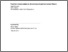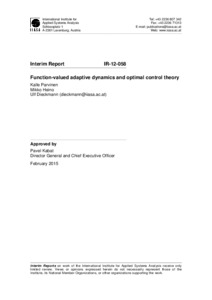# Function-valued adaptive dynamics and optimal control theory

, , & (2012). Function-valued adaptive dynamics and optimal control theory. IIASA Interim Report. IIASA, Laxenburg, Austria: IR-12-058Preview Text IR-12-058.pdf Download (352kB) | Preview

## Abstract

In this article we further develop the theory of adaptive dynamics of function-valued traits. Previous work has concentrated on models for which invasion fitness can be written as an integral in which the integrand for each argument value is a function of the strategy value at that argument value only. For this type of models of direct effect, singular strategies can be found using the calculus of variations, with singular strategies needing to satisfy Euler's equation with environmental feedback. In a broader, more mechanistically oriented class of models, the function-valued strategy affects a process described by differential equations, and fitness can be expressed as an integral in which the integrand for each argument value depends both on the strategy and on process variables at that argument value. In general, the calculus of variations cannot help analyzing this much broader class of models. Here we explain how to find singular strategies in this class of process-mediated models using optimal control theory. In particular, we show that singular strategies need to satisfy Pontryagin's maximum principle with environmental feedback. We demonstrate the utility of this approach by studying the evolution of strategies determining seasonal flowering schedules.

Item Type: Monograph (IIASA Interim Report) Evolution and Ecology (EEP) IIASA Import 15 Jan 2016 08:48 27 Aug 2021 17:22 https://pure.iiasa.ac.at/10218

### Actions (login required)View Item